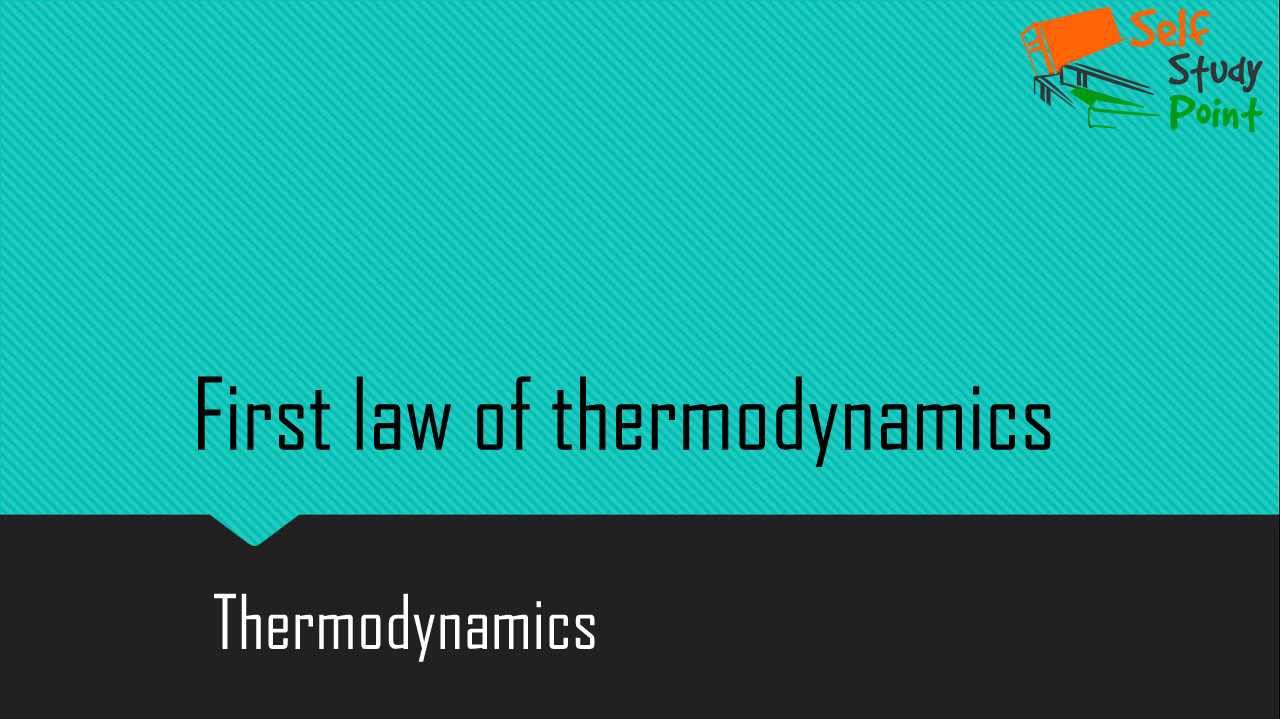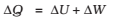First law of thermodynamics: The internal energy U of a system can change through two modes of energy transfer: heat and work. Let

ΔQ = Heat supplied to the system by the surroundings
ΔW = Work done by the system on the surroundings
ΔU = Change in internal energy of the system

The general principle of conservation of energy then implies thati.e. the energy (ΔQ) supplied to the system goes in part to increase the internal energy of the system (ΔU) and the rest of work on the environment (ΔW). This equation is known as the First Law of Thermodynamics.

Some important point:

1. Q is positive when heat is given to the system.
2. Q is negative when heat is taken from the system.
3. W is positive when system expands and does work on surroundings
4. In isothermal process, if the system undergoes any process in which ΔU=0 i.e., change in internal energy is zero then,
∆Q = ∆W
that is heat supplied to the system is used up entirely in doing work on the surroundings.
5. In an adiabatic process, no exchange of heat takes place, i.e., ∆Q = 0.
∆U = -∆W
6. In isochoric process, work done is zero, i.e., ∆W = 0
∆Q = ∆U
7. If the system is a gas in a cylinder with a movable piston, the gas in moving the piston does work. Since force is pressure times area, and area times displacement is volume, work done by the system against a constant pressure P is
∆W = P∆V
where ΔV is the change in volume of the gas. Thus, for this case,From above equation we see that first law of thermodynamics is a statement of conservation of energy stated as ‘ The energy put into the system equals the sum of the work done by the system and the change in internal energy of the system.’

Click on a star to rate it!

Average rating 0 / 5. Vote count: 0

No votes so far! Be the first to rate this post.

As you found this post useful...